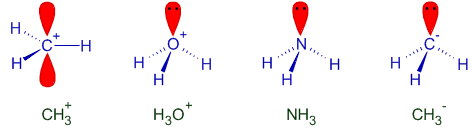< Previous question Next question >

# NUMBER OF ELECTRONS & ISOELECTRONIC SPECIES IIT JEE - NEET

## 1) The total number of electrons in one molecule of carbon dioxide is:

(IIT JEE 1979)

1) 22

2) 44

3) 66

4) 88

Logic:

Number of electrons in a molecule is equal to the sum of electrons in individual atoms in it.

# electrons in an atom is equal to its atomic number.

Solution:

In CO2, there are one carbon and two oxygen atoms and the number of electrons in them are:

In C ------> 6 electrons

In two O's -------> 2 x 8 electrons

Total in CO2 -------> 6 + (2 x 8) = 22 electrons.

Conclusion:

Correct option is '1'.

## Related questions

### 2) The total number of electrons that take part in forming the bonds in N2is:

(IIT JEE 1980)

1) 2

2) 4

3) 6

4) 8

Logic & Solution:

In N2 molecule, there is a triple bond between two nitrogen atoms. Hence the number of electrons that participate in bond formation is 6. Each nitrogen atom contributes three electrons for bond formation.

Conclusion:

Correct option is '3'.

### I) CH3+    II) H3O+    III) NH3    IV) CH3-

(IIT JEE 1993)

A) III & IV

B) I & II

C) I & III

D) II, III & IV

Logic & Solution:

 Species (sum of atomic numbers of each atom) - charge on the species I) CH3+ C + 3H - (+1) = 6 + 3(1) - 1 = 8 II) H3O+ 3H + O - (+1) = 3(1) + 8 - 1 = 10 III) NH3 N + 3H - (0) = 7 + 3(1) - 0 = 10 IV) CH3- C + 3H - (-1) = 6 + 3(1) +1 = 10

H3O+, NH3 and CH3- are not only isoelectronic but are also iso-structural with pyramidal shape.

However CH3+ is trigonal planar with one empty orbital perpendicular to this plane.Conclusion:

Correct option is 'D'.

## Homework

 < Previous question Next question >

Author: Aditya vardhan Vutturi